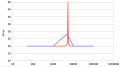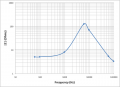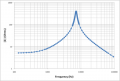# A graph of inductor in parallel with a capacitor

#### AlwaysNumber1

Joined Dec 4, 2016
52
Hello everyone!

Recently, I have been making an experiment to measure the module of impedance of an inductor in parallel with a capacitor.
The circuit that I used for it is shown below. Unknown impedance in this case is an inductor in parallel with a capacitor.

I need your help in several aspects of that graph:
1. Why the graph is curved unevenly to the right and to the left ?
2. On what factor does the sharpness of the graph depend ?
3. How could I possible improve my measurements ?

EVERY advice would be useful !

All the best,
Igor

#### wayneh

Joined Sep 9, 2010
17,273
Hello everyone!

Recently, I have been making an experiment to measure the module of impedance of an inductor in parallel with a capacitor.
The circuit that I used for it is shown below. Unknown impedance in this case is an inductor in parallel with a capacitor.

I need your help in several aspects of that graph:
1. Why the graph is curved unevenly to the right and to the left ?
2. On what factor does the sharpness of the graph depend ?
3. How could I possible improve my measurements ?

EVERY advice would be useful !

All the best,
Igor
It’s been a long time since I worked LC math but I don’t recall any reason to expect a symmetrical peak on a log-log chart.

The rise and fall of resonance is predictable from the math. It’s not all or nothing.

Throw in a little experimental variation and your results are probably quite reasonable.

Last edited:

#### OBW0549

Joined Mar 2, 2015
3,566
1. Why the graph is curved unevenly to the right and to the left ?
My guess is that it's probably an artifact of how your spreadsheet draws graphs, and a result of not having nearly enough data points in the immediate vicinity of the resonance frequency. In other words, most likely the curve looks distorted because it is drawn from a very sparse data set.

2. On what factor does the sharpness of the graph depend ?
The apparent lack of sharpness could be another consequence of not having enough data points around resonance. It could also be due to using an inductor with significant internal resistance, which lowers the Q of the resonant circuit and thus broadens the resonance peak.

3. How could I possible improve my measurements ?
Take more measurements at frequencies near the resonance point. I would suggest an additional 10 measurements on each side of the peak, concentrated near the peak.

•AlwaysNumber1

#### WBahn

Joined Mar 31, 2012
28,502
What is it that you are actually measuring and plotting?

Try plotting the curves for some LC combinations that have the same resonant frequency as your data set. Take into account a bit of resistance, particularly in the case of the inductor.

#### Veracohr

Joined Jan 3, 2011
767
Here's an example of the difference the number of data points makes. I used a spreadsheet to plot a parallel LC circuit impedance, in series with 20 ohms resistance, with two sets of data points. The red trace uses 701 data points from 50Hz to 100kHz, the blue trace uses 7 data points (at what I estimated the data points in your graph are). The calculations are the same, so you can see how much difference the resolution of the data makes.•wayneh

#### AlwaysNumber1

Joined Dec 4, 2016
52
My guess is that it's probably an artifact of how your spreadsheet draws graphs, and a result of not having nearly enough data points in the immediate vicinity of the resonance frequency. In other words, most likely the curve looks distorted because it is drawn from a very sparse data set.

The apparent lack of sharpness could be another consequence of not having enough data points around resonance. It could also be due to using an inductor with significant internal resistance, which lowers the Q of the resonant circuit and thus broadens the resonance peak.

Take more measurements at frequencies near the resonance point. I would suggest an additional 10 measurements on each side of the peak, concentrated near the peak.
Could you please share more theoretical information (for example, formulas) that describes the behaviour of the graph and why it is uneven ?

#### MrAl

Joined Jun 17, 2014
10,096
Hello everyone!

Recently, I have been making an experiment to measure the module of impedance of an inductor in parallel with a capacitor.
The circuit that I used for it is shown below. Unknown impedance in this case is an inductor in parallel with a capacitor.

I need your help in several aspects of that graph:
1. Why the graph is curved unevenly to the right and to the left ?
2. On what factor does the sharpness of the graph depend ?
3. How could I possible improve my measurements ?

EVERY advice would be useful !

All the best,
Igor

Hello,

You need to label your graph and say what you are graphing and what the axis is.

Here is a bit of data in the form of a graph for series and parallel RLC circuits. I have more but i need to know what you want to graph and what you want to see.#### AlwaysNumber1

Joined Dec 4, 2016
52
Hello,

You need to label your graph and say what you are graphing and what the axis is.

Here is a bit of data in the form of a graph for series and parallel RLC circuits. I have more but i need to know what you want to graph and what you want to see.
View attachment 141344View attachment 141344
I am making a graph of magnitude of impedance against frequency (log/log scale) and I am trying to understand the sharpness of the graph and the reason because of which it is uneven. Hope this helps

#### OBW0549

Joined Mar 2, 2015
3,566
Could you please share more theoretical information (for example, formulas) that describes the behaviour of the graph and why it is uneven ?
No, because I don't know the details of how your spreadsheet draws smoothed curves between data points.

Once again: you do not have enough data points. You need to include more measurements, especially right around the resonance point. Do that, and I think the resulting graph will make a lot more sense.

•AlwaysNumber1

#### BR-549

Joined Sep 22, 2013
4,928
Do you understand the difference between a linear scale and a logarithmic scale?

#### nsaspook

Joined Aug 27, 2009
11,292
Lets look at real components with a spectrum analyzer for a reality check.10uh parallel with 10000pf and 30 ohm resistor in series from the spectrum analyzer tracking generator.Using the internal tracking generator. X scale linear.X scale logRescaled amplitude over the frequency scan range.

Last edited:
•AlwaysNumber1 and wayneh

#### MrAl

Joined Jun 17, 2014
10,096
I am making a graph of magnitude of impedance against frequency (log/log scale) and I am trying to understand the sharpness of the graph and the reason because of which it is uneven. Hope this helps
Hi,

I think you need to define what you mean by "uneven" which is not a term usually used to define things like this.
"Uneven" is a term like "symmetrical" where you need to show what that is in reference to.

If you mean the end points are not the same, that may or may not be the case. In general though you should get a smooth curve.
If you look at my attachment in the previous thread, you'll see graphs that curve either upward or downward. They form a big "U" shape maybe upside down. For the series circuit, the amplitude at 2*w is the same as at w/2, and same at 4*w as at w/4, so there is some method to it.

Here is a graph of RLC impedance. It is shown for L=1 and C=1, then L=1/2 and C=2, then L=2 and C=1/2.
I dont know if you consider that "even" or "uneven".

#### Attachments

Last edited:
•AlwaysNumber1 and wayneh

#### OBW0549

Joined Mar 2, 2015
3,566
Do you understand the difference between a linear scale and a logarithmic scale?
I assume he does since he plotted his data, appropriately, on a log-log graph.

•AlwaysNumber1

#### nsaspook

Joined Aug 27, 2009
11,292
Rescaled amplitude ranges over 10kHz to 1MHz.Normalized generator output with no network. (notice scan generator blip here and on other displays)LC network reinstalled. X linearX logX scale log to 10MHz.

•AlwaysNumber1

#### OBW0549

Joined Mar 2, 2015
3,566
I did a little experiment with MS Excel to verify that the advice I gave here was valid:

Take more measurements at frequencies near the resonance point. I would suggest an additional 10 measurements on each side of the peak, concentrated near the peak.
After a bit of fiddling with component values, I ended up with a parallel resonant circuit with a 0.5 μF capacitor and a 1 mH inductor having 5 Ω internal resistance that fairly closely replicated the results shown on the TS's graph when plotting only his data points:Then I plotted a second graph using more data points, spaced closer together:In the above graph, the points closest to the resonance peak are at frequencies 1% apart from one another; those farther away are 10% apart; and the ones farthest away are 20% apart.

•AlwaysNumber1

#### AlwaysNumber1

Joined Dec 4, 2016
52
Thank you very much guys!

•OBW0549

#### MrAl

Joined Jun 17, 2014
10,096
Hi,

It's nice to see so many members taking an interest in this.

Here are three more graphs.

The first is a plot with different L and C so we can see how the values affect the sharpness.
The second is a plot with some resistance RL added in series with L.
The third is a plot with the parallel R removed (open) so there is just L and C and some resistance RL in series with L.

#### KL7AJ

Joined Nov 4, 2008
2,229
Hello everyone!

Recently, I have been making an experiment to measure the module of impedance of an inductor in parallel with a capacitor.
The circuit that I used for it is shown below. Unknown impedance in this case is an inductor in parallel with a capacitor.

I need your help in several aspects of that graph:
1. Why the graph is curved unevenly to the right and to the left ?
2. On what factor does the sharpness of the graph depend ?
3. How could I possible improve my measurements ?

EVERY advice would be useful !

All the best,
Igor
Your X axis is logarithmic; on a linear scale the curve will be symmetrical. The sharpness of the curve is determined by the Q, which is X/R. For any given inductor, the Q will increase with frequency, because the XL increases, while the R stays constant. (This is not strictly true at radio frequencies, because the inductor resistance may increase with skin effect)